﻿ PipeLay > Software Components & Operation > Defining a Line > Line Subcomponents > Material > Material Data Inputs

# Material Data Inputs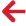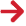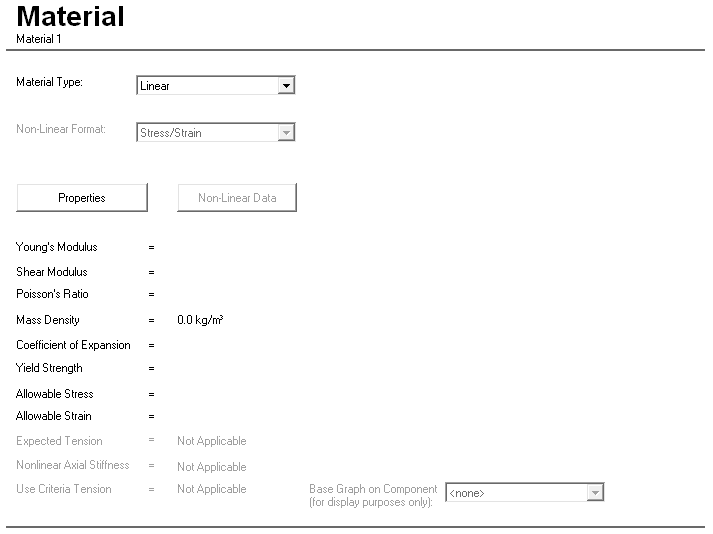Material Component Dialog

 Input Description Material Type: A drop-down list that allows you to select whether the material exhibits linear or non-linear behaviour. Non-Linear Format: A drop-down list that allows you to select the format of the non-linear material data. The options that are available are Stress/Strain, Moment/Curvature, or Ramberg-Osgood. The default is Stress/Strain. See Note (a). Properties Click on this button to display the Material Properties or Non-Linear Material Properties dialog, depending on the option you selected from the Material Type drop-down list. See the ‘Material Properties Dialog’ and ‘Non-Linear Material Properties Dialog’ sections for a detailed description of these dialogs. Non-Linear Data Click on this button to display a dialog in which you enter the non-linear material data. This button is only available when you select Non-Linear from the Material Type drop-down list. The dialog that is displayed depends on the option that you selected in the Non-Linear Format drop-down list. The non-linear data dialogs are described later in this article in the ‘Stress-Strain Curve Data Dialog’, ‘Moment/Curvature Curve Data Dialog’ and ‘Ramberg-Osgood Equation Data Dialog’ sections. Base Graph on Component: A drop-down list that allows you to select a pipe section or cable component. PipeLay will then display the material bending stiffness curve which results from the specified Ramberg-Osgood relationship for this component.  This input is for display purposes only and is optional.

Notes:

a)As it is not possible to convert moment-curvatures to stresses for the Moment-Curvature or Ramberg-Osgood material format, the predicted stresses for postprocessing output are computed as a product of the estimated bending or von Mises strains and the user specified Young's modulus. This can lead to an over estimation of stresses above the linear limit.

## Material Properties Dialog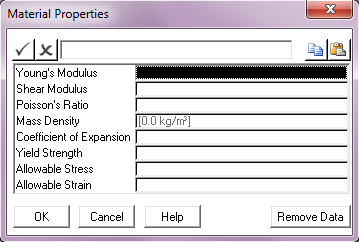Input Description Young’s Modulus: The Young’s Modulus (E) of the material. Units: [GPa] or [ksi] Shear Modulus: The Shear Modulus (G) of the material. This input is optional. The specification of Shear Modulus (G) is optional and, if omitted, is computed internally by PipeLay using the following relationship: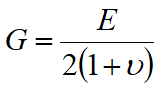where E is Young’s Modulus and ν is Poisson’s Ratio. Note that either G or ν must be a user-specified input. Units: [GPa] or [ksi] Poisson’s Ratio: The Poisson’s Ratio (ν) of the material. This input is optional. See the description above for Shear Modulus for more information. Mass Density: The mass density (ρ) of the material. This input is optional in certain circumstances. You have two options for specifying the weight in air and weight in water of the pipe sections and cables that comprise your model. The first option involves defining the material mass density here, and later specifying the diameter (and wall thickness, if applicable) when defining a Pipe Section or Cable component. From this PipeLay calculates the weight of material and the weight of water displaced (taking into account any additional buoyancy material you might specify for a Pipe Section component). Alternatively, you have the option to specify weight in air/unit length and weight in water/unit length directly when defining a Pipe Section or Cable component. If you exercise this latter option, then any Mass Density value you specify here is immaterial and unused, and input of this value can be considered optional in that circumstance. However, please note that the Material component can be referenced by many other pipeline sub-components in addition to the Pipe Section or Cable components, and for any of these a Mass Density value is required. Units: [kg/m3] or [slugs/ft3] Coefficient of Expansion: The coefficient of thermal expansion (α) of the material. This input is optional and has no function in the current version of PipeLay. It is retained for forward compatibility reasons. Units: [ºC-1] or [ºF-1] Yield Strength: The yield strength (σy) of the material. Units: [MPa] or [ksi] Allowable Stress: The allowable stress (σA), as a percentage of σy, of the material. Units: [%] Allowable Strain: The allowable strain (εΑ) of the material. Units: [%]

## Non-Linear Stress/Strain Material Properties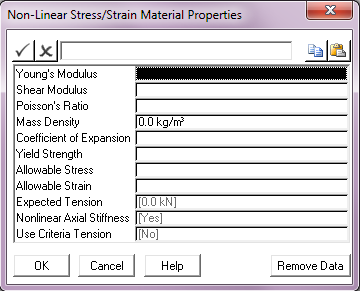Input Description Young’s Modulus: The secant modulus or slope of the non-linear material stress-strain curve at the origin (0,0). Units: [GPa] or [ksi] Shear Modulus: The Shear Modulus (G) of the material. This input is optional. The specification of Shear Modulus (G) is optional and, if omitted, is computed internally by PipeLay using the following relationship: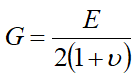where E is Young’s Modulus and ν is Poisson’s Ratio. Note that either G or ν must be a user-specified input. Units: [GPa] or [ksi] Poisson’s Ratio The Poisson’s Ratio (ν) of the material. This input is optional. See the description above for Shear Modulus for more information. Mass Density: The mass density (ρ) of the material. This input is optional in certain circumstances. You have two options for specifying the weight in air and weight in water of the pipe sections and cables that comprise your model. The first option involves defining the material mass density here, and later specifying the diameter (and wall thickness, if applicable) when defining a Pipe Section or Cable component. From this PipeLay calculates the weight of material and the weight of water displaced (taking into account any additional buoyancy material you might specify for a Pipe Section component). Alternatively, you have the option to specify weight in air/unit length and weight in water/unit length directly when defining a Pipe Section or Cable component. If you exercise this latter option, then any Mass Density value you specify here is immaterial and unused, and input of this value can be considered optional in that circumstance. However, please note that the Material component can be referenced by many other pipeline sub-components in addition to the Pipe Section or Cable components, and for any of these a Mass Density value is required. Units: [kg/m3] or [slugs/ft3] Coefficient of Expansion: The thermal coefficient of expansion (α) of the material. This input is optional and has no function in the current version of PipeLay. It is retained for forward compatibility reasons. Units: [ºC-1] or [ºF-1] Yield Strength: The yield strength (σy) of the material. Units: [MPa] or [ksi] Allowable Stress: The allowable stress (σΑ), as a percentage of σy, of the material. Units: [%] Allowable Strain: The allowable strain (εΑ) of the material. Units: [%] Expected Tension: The tension the material is expected to encounter during subsequent analysis. This tension value will reduce the bending capacity of any components referencing the material. Defaults to zero tension. Refer to Technical Note 7 for more details on the significance of this input. Units: [kN] or [kips] Nonlinear Axial Stiffness: An option to use linear (No) or non-linear (Yes) axial stiffness. The default is Yes to include non-linear axial stiffness. The option to use linear stiffness is provided to increase model stability/simplicity. Most PipeLay applications undergo linear axial deformation and so the use of a linear stiffness it worth consideration. Use Criteria Tension: A Yes or No (default) option to replace the Expected Tension value above with a maximum tension limit defined as part of criteria analysis. If Yes is selected and no tension criterion is defined on an analysis then the Expected Tension is used by default.

## Stress-Strain Curve Data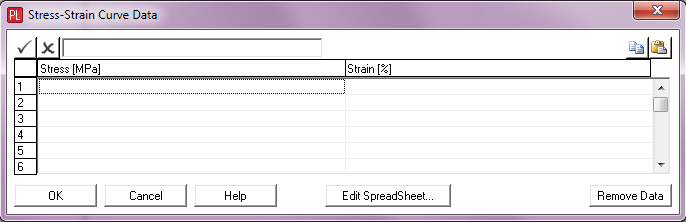Input Description Stress: Stress value defining a point on the material’s stress/strain curve. Units: [MPa] or [ksi] Strain: Corresponding value of strain at the same point. Units: [%] See Note (a).

Notes:

(a)Users should avoid sparsely spaced curve points at higher strain values where the curve has already plateaued. These isolated plateau points cause the conversion of the Stress-Strain data to Moment-Curvature to lose resolution around the yield point and so can lead to inaccurate predictions. Instead, users should focus their input curve points around the non-linear yield area, in order to get good resolution, and then let PipeLay extrapolate to the plateau region itself.

## Moment/Curvature Curve Data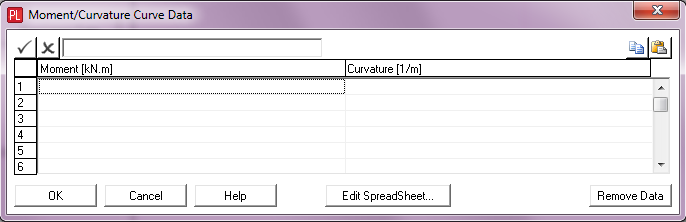Input Description Moment: Moment value defining a point on the material’s bending stiffness curve. Units: [kNm] or [kips.ft] Curvature: Corresponding curvature value. Units: [m-1] or [ft-1]

## Ramberg-Osgood Equation DataInput Description Ramberg Osgood Coefficient: The Ramberg-Osgood coefficient. The Ramberg-Osgood formulation defines a non-linear moment-curvature relationship using the following equations: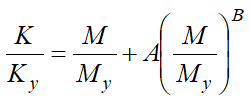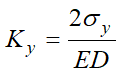;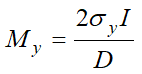where  A = Ramberg-Osgood coefficient,  B = Ramberg-Osgood exponent,  K = Pipe curvature,  Ky = Pipe curvature at yield stress,  M = Pipe bending moment,  My = Pipe bending moment at yield stress,  E = Young’s Modulus of the pipe,  D = External diameter of the pipe,  I = Moment of inertia of the pipe, and  σy = Yield stress of the pipe. Ramberg Osgood Exponent: The Ramberg-Osgood exponent. See the description above for Ramberg Osgood Coefficient for more information. Maximum Moment: The maximum moment at which the Ramberg-Osgood relationship is defined. This input is optional. When the Ramberg-Osgood option for non-linear material definition is invoked, a non-linear moment-curvature relationship is generated automatically by PipeLay. This is done for moments in the range from zero to the Maximum Moment you specify (positive and negative). If you do not explicitly define a Maximum Moment, PipeLay uses a value of 1.5* My. Units: [kNm] or [kips.ft]